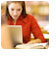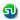### TANCET 2008 - Past year paper: Percent, Percent increase, Percentage and fractions: Ascent Education TANCET MBA Classes

 Home TANCET Classes GMAT Coaching Online GMAT Course CAT Classes TANCET Study Material
 TANCET '18 Classroom ProgramWeekend and weekday classes for TANCET MBA @ Chennai. At Nungambakkam and Velachery.Other Courses TANCET Correspondence Course CAT Classes & Courses GMAT Classes Chennai Online GMAT Course GRE Classes Chennai CBSE Math Online Tuition SAT Classes Other Links Ascent TANCET Toppers Testimonials CAT, TANCET Questions Careers @ Ascent Contact Us +91 44 4500 8484 +91 96000 48484 ascent@ascenteducation.com Postal Address Facebook / Twitter / Blog / Videos
You are here: Home  »  TANCET Prep Questions »  Percents, Fractions »   TANCET 2008 Question 14

# TANCET 2008 Quant Question 14 : Percent Increase

## Question

Joan started work 2 years ago. Her starting salary was half of Mike's salary at that time. Each year since then Joan and Mike have received a rise of 10% in their respective salary. What percentage (to the nearest percent) of Mike's current salary is Joan's current salary?
1. 45
2. 46
3. 48
4. 50
5. 220

Correct Answer : 50. Choice (4)

Let the salary of Mike 2 years ago be Rs.100
So, Joan's salary 2 years ago was Rs.50

Mikes present salary has seen two 10 percent increases. So has Joan's salary.
So, Mike's salary 1 year ago = 100 + 10% of 100 = 100 + 10 = 110.
Mike's present salary = 110 + 10% of 110 = 110 + 11 = 121.

Joan's salary 1 year ago = 50 + 10% of 50 = 50 + 5 = 55
Joan's present salary = 55 + 10% of 55 = 55 + 5.5 = 60.5

Joan's present salary of 60.5 = 50% of Mike's present salary of 121.

Level of difficulty: Easy

## CAT, XAT, TANCET Practice Questions and Answers : Listed Topicwise

 Number Theory Permutation Combination Probability Inequalities Geometry Mensuration Trigonometry Coordinate Geometry Percentages Profit Loss Ratio Proportion Mixtures Alligation Speed Time Distance Pipes Cisterns Interest Races Average, Mean AP, GP, HP Set Theory Clocks Calendars Algebra Function English Grammar General Awareness Data sufficiency TANCET Papers XAT PapersAdd to del.icio.usStumble Itdigg this